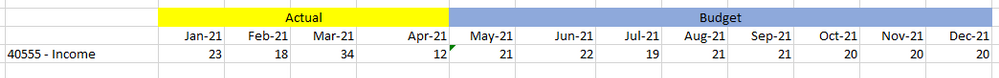# Allowing Time Sum function to accept 1st argument a functionI would like to ask if you may give timesum function ability to accept other function within timesum parentheses [ex: Timesum(previous(revenue),start period, end period)]. The main reason I asked this, I would like to calculate the average 3 month based on previous figure.[Excel example]

In meantime, I would also like to ask if there is some one can give me hand on this.

1